# Which of the following quantities have the dimensions of a distance?

Which of the following quantities have the dimensions of a distance?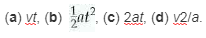The quantity with dimensions of a distance is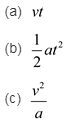(a) The dimensions of vt are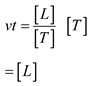This is the dimension for distance

(b)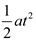The dimensions are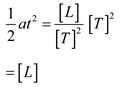This is the dimension for distance.

( c) The dimensions of 2at are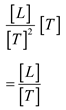This is not the dimension for distance.